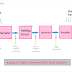ADC or Analog to Digital Converter is an electronic device or circuit that is used to convert the continuous analog electronic or electrical signal into a discrete digital signal. We know that when physical quantities such as sound, flow, temperature, etc are converted into an electrical or electronic signal it is generated in the form of an analog signal. But our processor works with digital signals only. Even there are so many advantages of a digital signal over an analog signal. So we need to convert the analog signal into a digital signal. There are huge applications of Analog to Digital Converter or ADC in electronic circuitry such as telecommunication circuits, automation circuits, measurement circuits, etc.

## Analog to Digital Converter (ADC) Block Diagram

Here, you can see the block diagram of ADC or Analog to Digital Converter.

The main blocks or parts are,

### Sampler

The sampler is a circuit that takes samples from the continuous analog signal according to its sample frequency. The sampling frequency is set according to the requirement. Basically, the sampler converts the continuous-time-continuous amplitude signal into a continuous amplitude-discrete time signal.

### Holding Circuit

The holding circuit does not convert anything it just holds the samples generated by the sampler circuit. It holds the first sample until the next sample comes from the sampler. Once the new sample comes from the sampler to the holding circuit it releases the old sample to its next block.

### Quantizer

The quantizer quantized the signal which means it converts the continuous amplitude-discrete time signal into discrete time-discrete amplitude signal. It breaks or splits the samples into small parts.

### Encoder

The encoder is the circuit that actually generates the digital signal into binary form. The output from the encoder is fed to the next circuitry. Here, is the end of the analog to a digital circuit.

## How Analog to Digital Converter(ADC) Works?

The working principle of the Analog to Digital converter is very simple. First, the input analog signal is fed to the sampler circuit. The sampler circuit takes samples from the analog signal with respect to discrete-time with the help of sample frequency. Now, the samples are held for some time in the holding circuit. After the holding circuit, the quantizer circuit receives the signal from the holding circuit and quantizes the signal. After the quantized signal is fed to the encoder circuit that actually generates the digital signal in binary form.

This was the basic concept of analog to digital conversion. There are so many factors is considered during the analog o digital conversion process. For example, a very important factor is Resolution. For analog signals, there is no maximum or minimum value that means the amplitude of the signal can reach an infinite value. On the other hand, for the digital signal, a maximum and minimum value are determined. When the amplitude is reached or crossed a particular voltage level it is to be considered as High and when the amplitude goes low under a particular voltage level it is being considered as low. But the ideal digital signal has zero amplitude when it is low or binary 0 and the amplitude will be maximum when it is high or binary 1.

Analog to Digital Converter (ADC) Block Diagram, WorkingReviewed by Author on 3/03/2022 Rating: 5